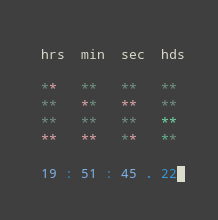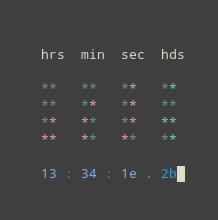# Playing With Bases

When I first got my Pebble Time smartwatch, I downloaded a couple of watchfaces that were right up my alley—the kind that made it really hard to tell the time! I was enthralled by BCD Minimalist, a clock using binary coded decimal format. Equally interesting, and great for practicing my 16 times table, was HexDateTime, which expresses the time and date in base 16 (hexadecimal), instead of base 10 (decimal).

So what is a base? You may not realize it, but the fact that the numerals 1 and 0 make ten (10) is only true in our base 10 system. To understand base systems, I think about our numerical system as follows: Each digit is multiplied by the base, raised to the power of the number of digits to the right of that digit. Let’s look at a random number, 251, as an example.

• in base 10 (25110)
• the first digit (2) is $2 * 10^2 = 200$
• the second digit (5) is $5 * 10^1 = 50$
• the third digit (1) is $1 * 10^0 = 1$
• if we sum those numbers, we get two hundred and fifty one, as we might expect.
• if, instead, we were dealing with base 16 (25116)
• the first digit (2) is $2 * 16^2 = 512$
• the second digit (5) is $5 * 16^1 = 80$
• the third digit (1) is $1 * 16^0 = 1$
• if we sum those numbers, we get five hundred and ninety three!

So, “wait a minute!", you say. “How can we possibly express ourselves in different bases if there are only ten digits in our number system?” Good question. In base 16, we can borrow from the alphabet to round-out our digits. Therefore, we use the regular digits from 0 to 9, as they are, and then borrow the letters a through f. Remember, 1016 is really sixteen.

On the weekend, I have a part-time job invigilating an international test. It can be hard to stay awake on a Saturday morning, so, as I’m sure you all do too, I pass the time doing base conversions by hand. (You do that too, right?) I got through the current year (201810 = 7e216), my birth date (04-04-7c5 in base 16), and some random numbers. One day, I sketched out how the BCD Minimalist watch face would look if each digit was in base 16 instead of base 10. I very soon realized that it wouldn’t change one bit (no pun intended), since 4 binary digits (binary, by the way, is base 2) is enough to express all numbers from 0 to 15 (i.e. from 0 to f in base 16). I thought about how nice it would be to have a desktop clock that combined my nerdy obsession with binary time visualization and the base 16 number system.

After a quick Google search, I found this very cool console-based binary clock written in Python by Brian Gajdos back in 2001. I took it for a spin and was pleased to see that, even at the end of 2017, the code still ran flawlessly. I stuck the applet on my desktop (KDE Plasma) through the Termoid Plasma widget.That was step one–I now had a BCD Minimalist-style clock on my desktop. I got into the code, and made a few hacky changes, which got me the end-result that I desired. A BCD clock with base 16 digits, that uses the full range (0-15) of the binary “LCDs”.The functions that I wrote were pretty hacky, but they did the trick of converting numbers up to fifteen (or higher in the case of b10()) from base 10 to base 16 or from base 16 to base 10.

def b10(s):
result = 0
for i in range(len(s)):
try:
except ValueError:
'b': '11',
'c': '12',
'd': '13',
'e': '14',
'f': '15'}.get(s[i]))
if (i != len(s)):
return result

def b16(n):
result = ''
remainder = n
for i in range(len(str(n)) - 1, 0, -1):
digit = int(remainder / 16)**i
digit = {'10': 'a',
'11': 'b',
'12': 'c',
'13': 'd',
'14': 'e',
'15': 'f'}.get(str(digit), str(digit))
result = result + digit
remainder = remainder % 16**i
if i == 1:
remainder = {'10': 'a',
'11': 'b',
'12': 'c',
'13': 'd',
'14': 'e',
'15': 'f'}.get(str(remainder), str(remainder))
result = result + remainder
return result


Take the number 14, for instance:

print(b16(14))

#> 0e


Can we revert that to base 10?

print(b10("0e"))

#> 14


Eventually, the simple number conversions from base 10 to base 16 and base 2 (binary) just didn’t cut it to keep me occupied any more, so I looked for a bigger challenge. I pretended that a certain code that we use at work, (let’s say “ac223”) was in base 16 and converted it to base 10 (ac22316 = 70505910). I wrote the calculation out three times by hand before I got it right (yep, I can write computer code but I can’t do grade-school math). Once I had finished with that problem, I started to think about other strings that I could convert to decimal. Being vain, I thought about my name, until I realized that only the first letter falls within the digits of the base 16 system. But, I thought, if we have 26 letters in the alphabet, and ten single-digit numerals, then we could, theoretically, express ourselves in bases up to 36 without needing to change the logic of the conversion that I laid out in the bullet points above. So, I set about writing it. I started with the easier conversion: base 10 to base $x$.

import string

def base_converter(input, base):

if base > 36:
raise ValueError("We don't have enough letters for that base.")
elif base > 10:
digits = [str(n) for n in list(range(10))] + [n for n in string.ascii_lowercase[:(base - 10)]]
else:
digits = [str(n) for n in list(range(base))]

places = 
i = 1

while places < input:
places.insert(0, base**i)
i += 1

result = ''
remainder = input

for place in places[1:]:
if place != 0:
times = remainder // place
remainder = remainder % place
result += (str(digits[times]))
else:
result += (str(digits[remainder]))

return(result)



What is 1000 in base 16?

print(base_converter(1000, 16))

#> 3e8


print(base_converter(1000, 2))

#> 1111101000


print(base_converter(27612362818, 36))

#> conoria


Great! I can express base 10 numbers in other bases, but can I do the reverse?

def base_reverter(input, base):

if base > 36:
raise ValueError("We don't have enough letters for that base.")
elif base > 10:
digits = [str(n) for n in list(range(10))] + [n for n in string.ascii_lowercase[:(base - 10)]]
else:
digits = [str(n) for n in list(range(base))]

places = 
i = 1

for j in range(len(input) - 1):
places.insert(0, base**i)
i += 1

result = 0
for digit in range(len(input)):
if (places[digit] != 0):
result += places[digit] * digits.index(input[digit])
else:
result += digits.index(input[digit])

return(result)


Let’s convert one thousand back from hexadecimal.

print(base_reverter('3e8', 16))

#> 1000


print(base_reverter('1111101000', 2))

#> 1000


Let’s double-check my math on the code I mentioned above.

print(base_reverter('ac223', 16))

#> 705059


In the interest of vanity…

print(base_reverter('conoria', 36))

#> 27612362818


Great! So I can convert one way or the other, but I can’t cross convert just yet. That is made easy with a quick wrapper function.

def base_xconverter(input, basein, baseout):
return(base_converter(base_reverter(input, basein), baseout))


Let’s see if we can make that thousand hop from base 16 straight to binary.

print(base_xconverter('3e8', 16, 2))

#> 1111101000


Now this is the kind of stuff I get excited over! While the application of this work up to this point is probably null, it might be fun to code “secret” research notes in a number for which only I know the base. This certainly wouldn’t stand up to a brute-force attack, but let’s try it anyway. We’ll “encrypt” the word “climate” into different bases. Since the true value never changes, we can convert between bases and then right back to base 36 with no lost information. We’ll start with base 36 (though we wouldn’t actually need all 26 letters of the alphabet in this case), spit it into base 20, 5, 2, and then back to 36.

print(base_xconverter('climate', 36, 20))

#> 1189a6836

print(base_xconverter('1189a6836', 20, 5))

#> 422130121420031

print(base_xconverter('422130121420031', 5, 2))

#> 11001100010100000010110011001000010

print(base_xconverter('11001100010100000010110011001000010', 2, 36))

#> climate


That wasn’t very effective encryption, was it? All a rival would need to know is the basein, which they could probably guess from the digits, and the baseout (that is, assuming they knew we were using base conversions to encrypt words). If we wanted to add an extra layer of security, we could add some secret steps. Let’s for instance, take our key word ‘climate’, and some secret password (‘password’), and make them interact in some secret way (addition).

value = base_reverter('climate', 36)
print(base_converter(encrypted, 36))

#> pnebizkr


Wow, look at that1 I guess I am a student in the U of T Pnebizkr Lab.

Encrypting our data would be no good if we can’t get it back. Let’s see the same steps in reverse:

value = base_reverter('pnebizkr', 36)
print(base_converter(decrypted, 36))

#> climate


There it is!

This blog post has been a fun exploration of different base systems, and how we can convert them in Python. If you enjoyed this post, I highly recommend the book “ Here’s Looking at Euclid” by Alex Bellos. Bellos takes a deep-dive into the world of math to reveal all of the quirky and curious things that you might never have known about numbers. It is even suitable for non-mathemeticians like myself.

This post was compiled on 2020-10-09 11:46:31. Since that time, there may have been changes to the packages that were used in this post. If you can no longer use this code, please notify the author in the comments below.

Packages Used in this post
sessioninfo::package_info(dependencies = "Depends")

#>  package     * version    date       lib source
#>  assertthat    0.2.1      2019-03-21  RSPM (R 4.0.0)
#>  cli           2.0.2      2020-02-28  RSPM (R 4.0.0)
#>  crayon        1.3.4      2017-09-16  RSPM (R 4.0.0)
#>  digest        0.6.25     2020-02-23  RSPM (R 4.0.0)
#>  evaluate      0.14       2019-05-28  RSPM (R 4.0.0)
#>  fansi         0.4.1      2020-01-08  RSPM (R 4.0.0)
#>  fs            1.5.0      2020-07-31  RSPM (R 4.0.2)
#>  glue          1.4.2      2020-08-27  RSPM (R 4.0.2)
#>  htmltools     0.5.0      2020-06-16  RSPM (R 4.0.1)
#>  hugodown      0.0.0.9000 2020-10-08  Github (r-lib/[email protected])
#>  jsonlite      1.7.1      2020-09-07  RSPM (R 4.0.2)
#>  knitr         1.30       2020-09-22  RSPM (R 4.0.2)
#>  lattice       0.20-41    2020-04-02  RSPM (R 4.0.0)
#>  magrittr      1.5        2014-11-22  RSPM (R 4.0.0)
#>  Matrix        1.2-18     2019-11-27  RSPM (R 4.0.0)
#>  rappdirs      0.3.1      2016-03-28  RSPM (R 4.0.0)
#>  Rcpp          1.0.5      2020-07-06  RSPM (R 4.0.2)
#>  reticulate    1.16       2020-05-27  RSPM (R 4.0.2)
#>  rlang         0.4.7      2020-07-09  RSPM (R 4.0.2)
#>  rmarkdown     2.3        2020-06-18  RSPM (R 4.0.1)
#>  sessioninfo   1.1.1      2018-11-05  RSPM (R 4.0.0)
#>  stringi       1.5.3      2020-09-09  RSPM (R 4.0.2)
#>  stringr       1.4.0      2019-02-10  RSPM (R 4.0.0)
#>  withr         2.3.0      2020-09-22  RSPM (R 4.0.2)
#>  xfun          0.18       2020-09-29  RSPM (R 4.0.2)
#>  yaml          2.2.1      2020-02-01  RSPM (R 4.0.0)
#>
#>  /home/conor/Library
#>  /usr/local/lib/R/site-library
#>  /usr/local/lib/R/library


1. I hope it goes without saying that you should probably rely on trusted encryption methods instead of some silly base conversions if you have truly sensitive data. This was just a toy example for fun. ↩︎##### Conor I. Anderson
###### PhD Candidate, Climate Lab

Conor is a PhD candidate in the Department of Physical and Environmental Sciences at the University of Toronto Scarborough (UTSC).